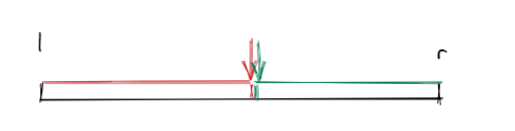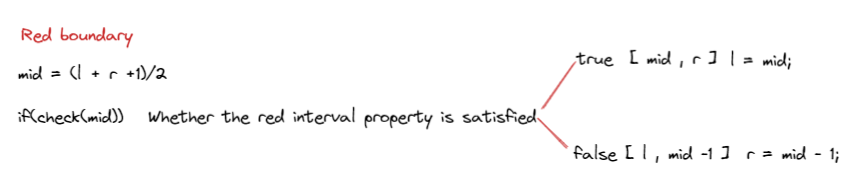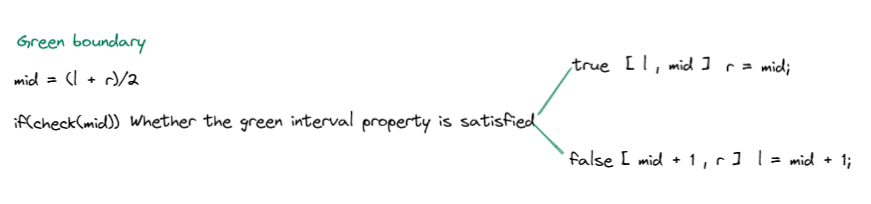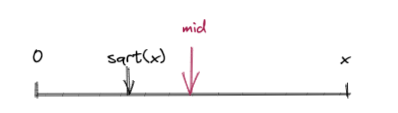# 算法基础（三）| 二分图解及代码模板

• 2022 年 9 月 22 日
山东
• 本文字数：1508 字

阅读完需：约 5 分钟

⭐写在前面的话：本系列文章旨在复习算法刷题中常用的基础算法与数据结构，配以详细的图例解释，总结相应的代码模板，同时结合例题以达到最佳的学习效果。本专栏面向算法零基础但有一定的 C++基础的学习者。若 C++基础不牢固，可参考：10min快速回顾C++语法，进行语法复习。

🔥本文已收录于算法基础系列专栏： 算法基础教程 免费订阅，持续更新。# 二分

## 整数二分• 假设 mid = (l + r ) / 2 ；如果是 l = r - 1;那么下取整后 mid = l ，会陷入死循环。### 例题：数的范围

1≤n≤1000001≤q≤100001≤k≤10000

6 31 2 2 3 3 4345

3 45 5-1 -1

### 代码模板

#include<bits/stdc++.h>using namespace std;const int N = 100010;int m ,n ;int q[N];int main(){    scanf("%d%d", &n, &m);    for(int i = 0; i < n ; i++)scanf("%d",&q[i]);        while( m --)    {        int x;         scanf("%d", &x);                int l = 0 , r = n - 1;        while( l < r)        {            int mid = l + r >> 1;            if(q[mid] >= x)r = mid;            else l = mid + 1;        }        //上面二分出来的是第一个满足大于等于x的数，如果没有x，则是大于x的数。        if(q[l] != x)cout << "-1 -1" <<endl;        //对该数进行判断，如果不满足，则返回-1-1。        else        {            //找到最后一个x的位置            cout << l << ' ';                        int l = 0, r = n - 1;            while(l < r)            {                int mid = l + r + 1 >> 1;                if(q[mid] <= x)l = mid;                else r = mid - 1;            }            cout << l << endl;        }    }}

## 浮点数二分

### 例题：开平方

−10000≤n≤10000

4

2.000000

### 代码模板#include<bits/stdc++.h>using namespace std;int main(){    double x;    cin >> x;        double l = 0, r =x ;    while(r - l > 1e-8)    {        double mid = (l + r)/2;        if(mid * mid >= x)r = mid;        else l = mid ;    }    printf("%lf", l);    return 0;}

while(r - l > 1e-8)

for(int i = 0; i < 100 ; i++);## 评论